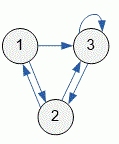Problems

# Count the number of edges

Time limit 1 second
Memory limit 128 MiB

The directed graph is given with an adjacency matrix. Find the number of edges in a graph.

## Input data

The first line contains the number of vertices in the graph n\:(1 \le n \le 100). Then given n lines with n numbers 0 or 1 — the adjacency matrix of the graph.

## Output data

Print the number of edges in a graph.## Examples

Input example #1
3
0 1 1
1 0 1
0 1 1

Output example #1
6